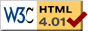### Journée-séminaire de combinatoire

#### (équipe CALIN du LIPN, université Paris-Nord, Villetaneuse)

Le 01 février 2022 à 14h00 en B107 & BBB visioconférence, Alantha Newman nous parlera de : Voting algorithms for unique games on complete graphs

Résumé : An approximation algorithm for a Constraint Satisfaction Problem is called robust if it outputs an assignment satisfying a $(1 - f(\epsilon))$-fraction of the constraints on any $(1-\epsilon)$-satisfiable instance, where the loss function $f$ is such that $f(\epsilon) \rightarrow 0$ as $\epsilon \rightarrow 0$. Moreover, the runtime of the algorithm should not depend in any way on $\epsilon$. We present such an algorithm for the Unique Games Problem on complete graphs with $q$ labels. Specifically, the loss function is $f(\epsilon) = (\epsilon + c_\epsilon \epsilon^2)$, where $c_\epsilon$ is a constant depending on $\epsilon$ such that $\lim_{\epsilon \rightarrow 0} c_\epsilon = 16$. The runtime of our algorithm is $O(qn^3)$ (with no dependence on $\epsilon$) and can run in time $O(qn^2)$ using a randomized implementation with a slightly larger constant $c_\epsilon$. Our algorithm is combinatorial and uses voting to find an assignment. We prove NP-hardness (using a randomized reduction) for Unique Games on complete graphs even in the case where the constraints form a cyclic permutation, which is also known as Min-Linear-Equations-mod-q on complete graphs. This is joint work with Antoine Meot, Moritz Muehlenthaler and Arnaud de Mesmay.

[Slides.pdf] [arXiv] [vidéo]

 Dernière modification : Monday 24 January 2022Contact pour cette page : Cyril.Banderier at lipn.univ-paris13.fr